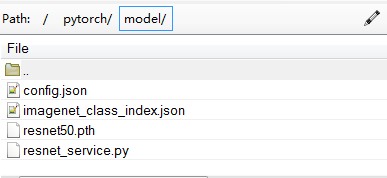2020年6月更新，调整代码的组成结构，使其更加通用，方便使用，更加整洁，添加模型蒸馏的方法。

lxztju/pytorch_classificationgithub.com2020年4月26更新：cnn svm knn pytorch

2020年4月更新

• 基础功能利用pytorch实现图像分类
• 包含带有warmup的cosine学习率优化
• 多模型融合预测，加权与投票融合

https://github.com/lxztju/pytorch_classificationgithub.com

lxztju/densenet-pytorchgithub.com3、由于在分类任务中，一般需要读取预训练权重进行预处理，加快网络的收敛。但是，我们一般的分类的类别数目不尽相同，因此许啊哟读入前边几层，最后一层的参数利用初始化函数进行初始化，这里介绍了读取部分预训练模型参数的方法，可以很好的理解pytorch保存的模型为字典格式

4、介绍多gpu并行训练的方式

5、介绍多gpu训练的模型保存参数与单gpu训练保存的模型参数的不同点，以及加载多gpu模型时需要对模型的键进行处理，去掉‘module’

6、在进行预测评估时，使用eval()函数

## 1、准备自己的数据集

``````##
train_transforms = transforms.Compose([    transforms.RandomRotation(10),    transforms.RandomResizedCrop(224),    transforms.RandomHorizontalFlip(),    transforms.ToTensor(),    transforms.Normalize((.5, .5, .5), (.5, .5, .5))])
train_dir = cfg.TRAIN_DATASET_DIR
train_datasets = datasets.ImageFolder(train_dir, transform=train_transforms)

## 3、训练代码

github代码中的train.py

``````##读入下载的预训练的权重字典

###去掉全链接层的权重，
#由于我们一般不会直接使用，imagenet的1000类，因此，我们需要更换网络最后的全链接层
#因此我们需要将前边几层的参数保存，最后一层重新初始化
#定义一个新的字典，将原始的参数字典，对应保存与更改
from collections import OrderedDict
new_state_dict = OrderedDict()

for k,v in state_dict.items():
# print(k)  #打印预训练模型的键，发现与网络定义的键有一定的差别，因而需要将键值进行对应的更改，将键值分别对应打印出来就可以看出不同，根据不同进行修改
#torchvision中的网络定义，采用了正则表达式，来更改键值，因为这里简单，没有再去构建正则表达式
# 直接利用if语句筛选不一致的键
###修正键值的不对应
if k.split('.') == 'features' and (len(k.split('.')))>4:
k = k.split('.')+'.'+k.split('.')+'.'+k.split('.')+'.'+k.split('.')[-3] + k.split('.')[-2] +'.'+k.split('.')[-1]
# print(k)
else:
pass
##最后一层的全连接层，进行初始化
if k.split('.') == 'classifier':
if k.split('.')[-1] == 'weights':
v = nn.init.kaiming_normal(model.state_dict()[k], mode='fan_out')
else:
model.state_dict()[k][...] = 0.0
v = model.state_dict()[k][...]
else:
pass
##得到新的与定义网络对应的预训练参数
new_state_dict[k] = v
##导入网络参数

##进行多gpu的并行计算
if args.ngpu:
model = nn.DataParallel(model,device_ids=list(range(args.ngpu)))
print("initialize the network done")

loss_func = nn.CrossEntropyLoss()``````

``````##训练max——epoch个epoch
for epoch in range(args.max_epoch):
model.train()  ##在进行训练时加上train()，测试时加上eval()
##在测试时加上eval()会将BN与Dropout的进行固定
batch = 0

# print(batch_labels)
# print(torch.cuda.is_available())
average_loss = 0
train_acc = 0

##在pytorch0.4之后将Variable 与tensor进行合并，所以这里不需要进行Variable封装
if torch.cuda.is_available():
batch_images, batch_labels = batch_images.cuda(),batch_labels.cuda()
out = model(batch_images)
loss = loss_func(out,batch_labels)

#    print(loss)
average_loss = loss
prediction = torch.max(out,1)
# print(prediction)

train_correct = (prediction == batch_labels).sum()
##这里得到的train_correct是一个longtensor型，需要转换为float
# print(train_correct.type())
train_acc = (train_correct.float()) / args.batch_size
#       print(train_acc.type())

loss.backward()  #loss反向传播
optimizer.step()  ##梯度更新

batch+=1
print("Epoch: %d/%d || batch:%d/%d average_loss: %.3f || train_acc: %.2f"
%(epoch, args.max_epoch, batch, max_batch, average_loss, train_acc))

##每10epoch保存一次模型
if epoch%10 ==0 and epoch>0:
torch.save(model.state_dict(), args.save_folder+'/'+'dense169'+'_'+str(epoch)+'.pth')
``````

## 4、测试网络模型效果

github代码中eval.py

``````###读取网络模型的键值对
trained_model = cfg.TRAINED_MODEL

# create new OrderedDict that does not contain `module.`
##由于之前的模型是在多gpu上训练的，因而保存的模型参数，键前边有‘module’，需要去掉，和训练模型一样构建新的字典
from collections import OrderedDict
new_state_dict = OrderedDict()
for k, v in state_dict.items():
name = k[7:] # remove `module.`
else:
name = k
new_state_dict[name] = v

##进行模型测试时，eval（）会固定下BN与Dropout的参数
model.eval()
eval_acc = 0.0

# print(batch_labels)
if torch.cuda.is_available():
batch_images, batch_labels = batch_images.cuda(), batch_labels.cuda()
##在pytorch0.4的版本之前，使用Variable封装，里边采用volatile=True放置进行反传训练

out = model(batch_images)

prediction = torch.max(out, 1)
num_correct = (prediction == batch_labels).sum()
eval_acc += num_correct
print(eval_acc)
print(' Accuracy of batch : {:.6f}'.format((eval_acc.float()) / (len(val_datasets))))
``````

## 5、单张图片结果预测

github代码中的predict.py

``````##labels_to_classes为与输出结果对应的字典，例如：{'0','flower'}
labels2classes = cfg.labels_to_classes

###读取网络模型的键值对
trained_model = cfg.TRAINED_MODEL

# create new OrderedDict that does not contain `module.`
##由于之前的模型是在多gpu上训练的，因而保存的模型参数，键前边有‘module’，需要去掉，和训练模型一样构建新的字典
from collections import OrderedDict
new_state_dict = OrderedDict()
for k, v in state_dict.items():
name = k[7:] # remove `module.`
else:
name = k
new_state_dict[name] = v

##进行模型测试时，eval（）会固定下BN与Dropout的参数
model.eval()

if torch.cuda.is_available():
batch_images, batch_labels = batch_images.cuda(), batch_labels.cuda()
##在pytorch0.4的版本之前，使用Variable封装，里边采用volatile=True放置进行反传训练

out = model(batch_images)

prediction = torch.max(out, 1)
print(labels2classes[str(prediction)]``````

## 6、华为云modelarts部署

deployment中的代码主要参考这个，主要应用于将模型部署到华为云modelarts上，这里就先不叙述了

ModelArts平台运行PyTorch模型_ModelArts_EI企业智能_热门产品_华为云bbs.huaweicloud.com